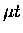Next: Spin flip Up: Muonic processes Previous: Muonic processes

### Elastic scattering

 F=0,1 F=0,1 (66)

 F=0,1 F=0,1 F=0,1 F=0,1 (67)

Elastic scattering cross sections for the symmetric (Eq. 5.6) and asymmetric (Eq. 5.7) cases were taken from Bracci et al. , and Chiccoli et al. , respectively. These are known as Nuclear Atlas cross sections,'' since the calculations assume scattering with the bare nucleus, as opposed to the atom or molecule. For the symmetric case, differential cross sections published by Melezhik and Wozniak  were used to give the final angular distributions. Similar differential cross sections for asymmetric collisions have not been published yet, but were calculated by Wozniak  and used as inputs to the simulation.

There are more recent calculations which take into account the molecular effects  as well as solid state effects . However, not all these cross sections are available in differential forms as of the writing of this thesis. Because we are interested in the transport of muonic atoms, it is essential to use differential cross sections, and therefore we chose to use the above nuclear'' cross sections which are available in differential form. For energies above about 0.2 eV, the molecular and solid state effects are small, therefore for the resonant molecular formation atenergies of 0.5 - 2 eV, the use of nuclear cross sections should be a good approximation. At lower energies, however, solid state processes become increasingly important.Next: Spin flip Up: Muonic processes Previous: Muonic processes